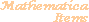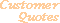Home Older Mathematica Items

Scientific Arts

# Single Electron Atom Wave Functions

Page 1 | Page 2 | Page 3

## Wave Functions

The angular portion of the wave function for a single electron atom is given by the spherical harmonic denoted (in spherical polar coordinates) by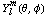. Mathematica provides this as the built-in function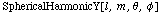.

The radial wave function is proportional to a generalized Laguerre polynomial,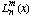, which is also provided in Mathematica by the function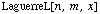.  Mathematica's definition of the generalized Laguerre polynomial follows the normalization convention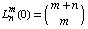. This is different than the convention used in some quantum mechanics texts (Landau and Lifshitz, for example).

Here is a program for the radial portion of the wave function. It has the conditional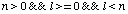to check to see that the quantum numbers satisfy the usual constraints.

``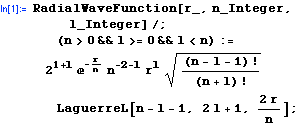``

We now make use of the Utilities`Notation` standard add-on package to give a definition for the radial wave function that looks a bit more like the conventional notation. The radial wave function will have the alternative form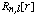.

``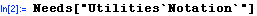``

Next use the Notation palette (which appears after the package is loaded) to place the template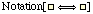, fill it in as follows, and execute the result. (Note that it is necessary to use the palette to carry out these tasks since the palette automatically inserts the appropriate code into the notebook to enable the notation. The shading in the following expression is inserted by the notation palette to remind you of this fact.)

``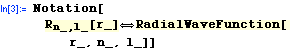``

Now the new notation can be used. For example, here is the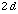radial wave function.

``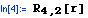``
`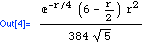`

Of course this is just the same as,

``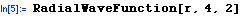``
`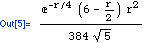`

We use the Notation function again to invoke the traditional textbook notation for the spherical harmonics.

``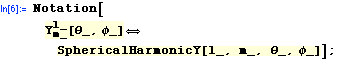``

For example,

``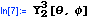``
`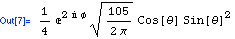`

The full wave function follows. We give it in a way that accepts two different patterns for its spatial arguments. In the first, with the form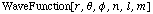, the expression in spherical polar coordinates is used. In the second, when the coordinates are presented as a list in the form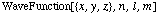the expression in Cartesian coordinates is utilized.

``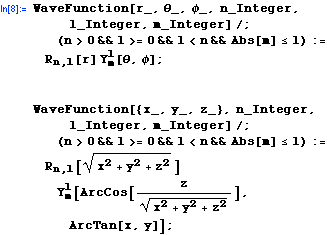``

Again we use the Notation function as earlier to create the traditional textbook notation. Two forms are given that accept the two different patterns for the arguments.

````

Here is the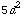wave function in spherical polar coordinates.

``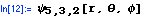``
`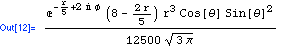`

Here it is in Cartesian coordinates.

``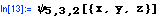``
`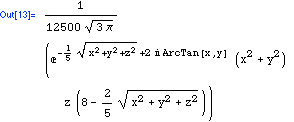`

The following is a palette to aid in the input of the notation (select the cell bracket and choose "Generate Palette From Selection" from the "File" menu to create the palette window).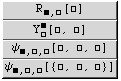## Visualization

 For further information on our services send email to info@scientificarts.com . Contents of this web site Copyright © 1999-2011, Scientific Arts, LLC.
 s